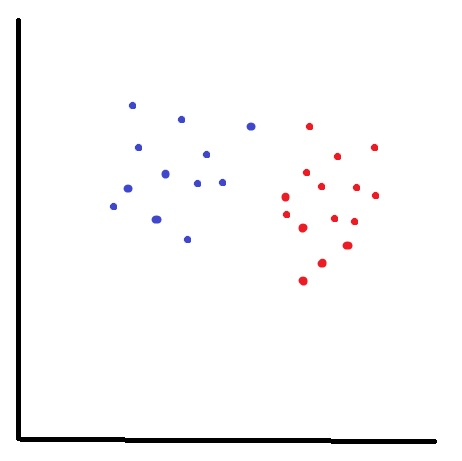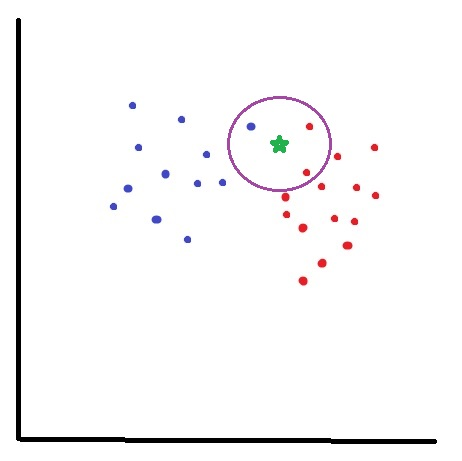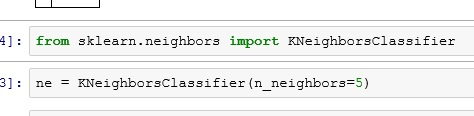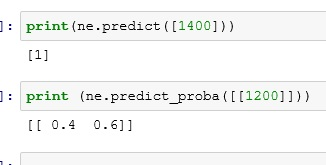# Python: K Nearest Neighbor

K Nearest Neighbor (Knn) is a classification algorithm. It falls under the category of supervised machine learning. It is supervised machine learning because the data set we are using to “train” with contains results (outcomes). It is easier to show you what I mean.

Here is our training set: logi

Let’s import our set into PythonThis data set contains 42 student test score (Score) and whether or not they were accepted (Accepted) in a college program.  It is the presence of the Accepted column that makes supervised machine learning possible. Knowing the outcomes of past events, we can create a prediction model for future events. So you could use the finished model to predict whether someone will be accepted based on their test score.

### So how does Knn work?

Look at the chart below. Imagine this represents our data set. Each blue dot is accepted (1) while each red dot is not(0).What if I want to know about my new data point (green star)? Is it a 1 or a 0?I start by choosing a neighbor count – in this example I will choose 3, and I find the 3 nearest neighbors to my new point.

Let’s look at the results, I have 2 red(0) and 1 blue(1). Using basic probability, I am 67% (2/3) certain that you will not get in.## Now, let’s code it!

First we need to separate our data into 2 dataframes: Our training set X (Score) and our target set y (Accepted)

df.pop() removes the Accepted column from your dataframe and places it in a newly created one.### Import sklearn

sklearn is a massive library of machine learning algorithms available for Python. Today we are going to use KNeighborsClassfier

So below imported KNeighborsClassifier from sklearn.neighbors

Next I set my neighbor count to 5. You can experiment with other numbers and see how works out for you. Setting the neighbor count is something you kind of have to develop a feel for.Now let’s fit the model with our training set(X) and target set(y)Now we can use our model to make predictions.

ne.predict() will return 1 or 0 – (Accepted or Not)

while ne.predict_proba() will return a probability range. Results below read as (40% change of not Accepted(0), 60% chance of Accepted(1))So there you go, you have now built a prediction model using K Nearest Neighbor.